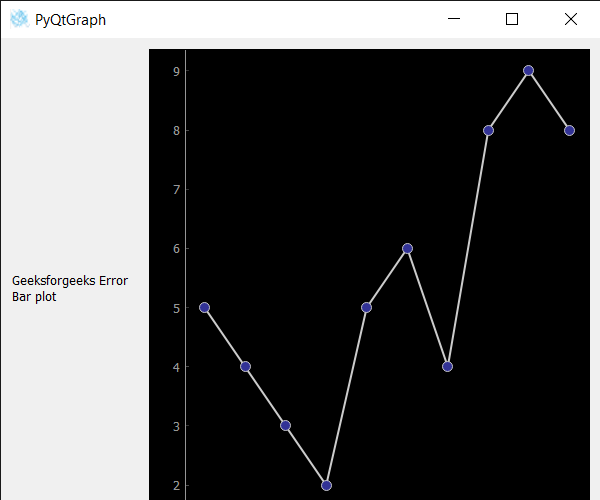Related Articles
PyQtGraph – Setting Visibility Property of Error Bar Graph
• Last Updated : 24 Oct, 2020

In this article, we will see how we can set the visibility property of the error bar graph in the PyQtGraph module. The PyQtGraph is a graphics and user interface library for Python that provides functionality commonly required in designing and science applications. Its primary goals are to provide fast, interactive graphics for displaying data (plots, video, etc.). Error bars are graphical representations of the variability of data and used on graphs to indicate the error or uncertainty in a reported measurement. They give a general idea of how precise a measurement is, or conversely, how far from the reported value the true value might be. By default, error bar graph is visible although we can change this property to make it invisible.

We can create a plot window and create error bar graph on it with the help of commands given below:

```# creating a pyqtgraph plot window
plt = pg.plot()

# creating a error bar item object
error = pg.ErrorBarItem(x=x, y=y, top=top, bottom=bottom, beam=0.5)
```

In order to do this, we use setVisible() method with the error bar item object

Syntax : error.setVisible(False)
Argument : It takes bool as argument
Return : It returns None

Below is the complete program to set visibility property of error graph:

## Python3

 `# importing Qt widgets``from` `PyQt5.QtWidgets ``import` `*`` ` `# importing system``import` `sys`` ` `# importing numpy as np``import` `numpy as np`` ` `# importing pyqtgraph as pg``import` `pyqtgraph as pg``from` `PyQt5.QtGui ``import` `*``from` `PyQt5.QtCore ``import` `*`` ` `from` `collections ``import` `namedtuple`` ` ` ` `class` `Window(QMainWindow):`` ` `    ``def` `__init__(``self``):``        ``super``().__init__()`` ` `        ``# setting title``        ``self``.setWindowTitle(``"PyQtGraph"``)`` ` `        ``# setting geometry``        ``self``.setGeometry(``100``, ``100``, ``600``, ``500``)`` ` `        ``# icon``        ``icon ``=` `QIcon(``"skin.png"``)`` ` `        ``# setting icon to the window``        ``self``.setWindowIcon(icon)`` ` `        ``# calling method``        ``self``.UiComponents()`` ` `        ``# showing all the widgets``        ``self``.show()`` ` `    ``# method for components``    ``def` `UiComponents(``self``):`` ` `        ``# creating a widget object``        ``widget ``=` `QWidget()`` ` `        ``# creating a label``        ``label ``=` `QLabel(``"Geeksforgeeks Error Bar plot"``)`` ` `        ``# setting minimum width``        ``label.setMinimumWidth(``130``)`` ` `        ``# making label do word wrap``        ``label.setWordWrap(``True``)`` ` `        ``# setting configuration options``        ``pg.setConfigOptions(antialias``=``True``)`` ` `        ``# creating x-axis values``        ``x ``=` `np.array([``1``, ``2``, ``3``, ``4``, ``5``, ``6``, ``7``, ``8``, ``9``, ``10``])`` ` `        ``# creating y-axis values``        ``y ``=` `np.array([``5``, ``4``, ``3``, ``2``, ``5``, ``6``, ``4``, ``8``, ``9``, ``8``])`` ` `        ``# creating upper bound values``        ``top ``=` `np.array([``2``, ``2``, ``2``, ``2``, ``2``, ``2``, ``2``, ``2``, ``2``, ``2``])`` ` `        ``# creating lower bound values``        ``bottom ``=` `np.array([``2``, ``2``, ``2``, ``2``, ``2``, ``2``, ``2``, ``2``, ``2``, ``2``])`` ` `        ``# creating a plot window``        ``plt ``=` `pg.plot()`` ` `        ``# creating a error bar item``        ``error ``=` `pg.ErrorBarItem(beam``=``0.5``)`` ` `        ``# setting data to error bar item``        ``error.setData(x``=``x, y``=``y, top``=``top, bottom``=``bottom)`` ` `        ``# adding error bar item to the plot window``        ``plt.addItem(error)`` ` `        ``# plotting the data on plot window``        ``plt.plot(x, y, symbol``=``'o'``, pen``=``{``'color'``: ``0.8``, ``'width'``: ``2``})`` ` `        ``# Creating a grid layout``        ``layout ``=` `QGridLayout()`` ` `        ``# minimum width value of the label``        ``label.setMinimumWidth(``130``)`` ` `        ``# setting this layout to the widget``        ``widget.setLayout(layout)`` ` `        ``# adding label in the layout``        ``layout.addWidget(label, ``1``, ``0``)`` ` `        ``# plot window goes on right side, spanning 3 rows``        ``layout.addWidget(plt, ``0``, ``1``, ``3``, ``1``)`` ` `        ``# setting this widget as central widget of the main widow``        ``self``.setCentralWidget(widget)`` ` `        ``# setting visibility property of error bar item``        ``error.setVisible(``False``)`` ` ` ` `# create pyqt5 app``App ``=` `QApplication(sys.argv)`` ` `# create the instance of our Window``window ``=` `Window()`` ` `# start the app``sys.exit(App.``exec``())`

Output:Attention geek! Strengthen your foundations with the Python Programming Foundation Course and learn the basics.

To begin with, your interview preparations Enhance your Data Structures concepts with the Python DS Course.

My Personal Notes arrow_drop_up